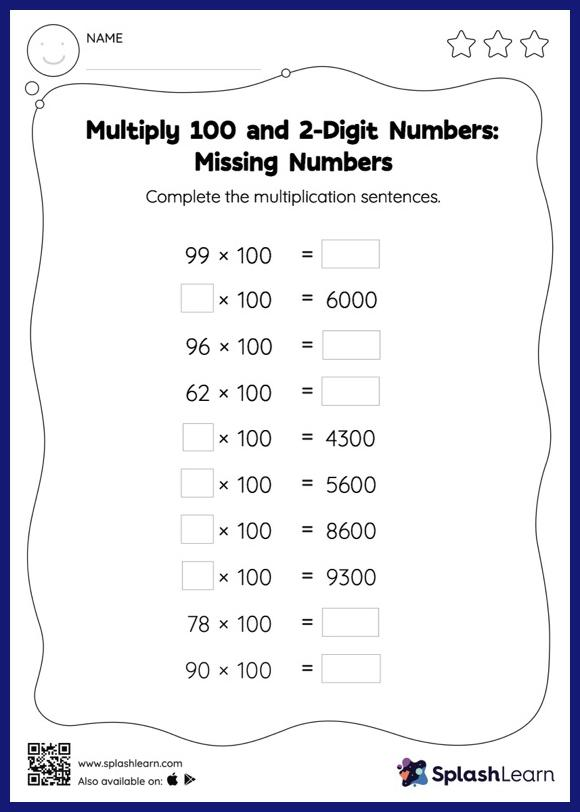# Multiply 100 and 2-Digit Numbers: Missing Numbers Worksheet

Home > Multiply 100 and 2-Digit Numbers: Missing NumbersChildren practice multiplying 100 and 2-digit numbers with this worksheet. Students love it when they get to multiply numbers by 10, 100, or their multiples. They use the pattern of zeros to find the product. In multiply 100 and 2-digit numbers worksheet, they use the same concept to find the missing number in multiplication sentences. In each problem, the numbers are laid out in the horizontal format. Students should try to use different strategies involving composing and decomposing numbers to solve these problems. This will help them develop flexibility and fluency.